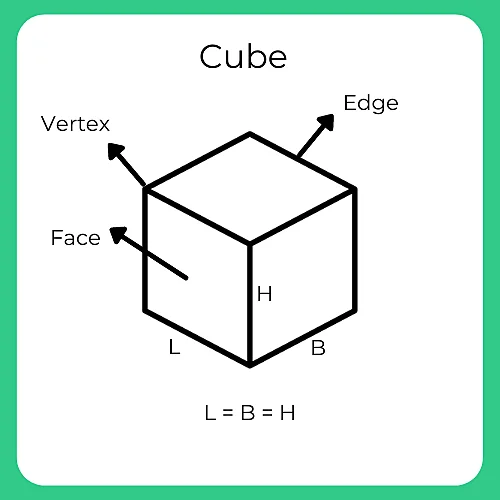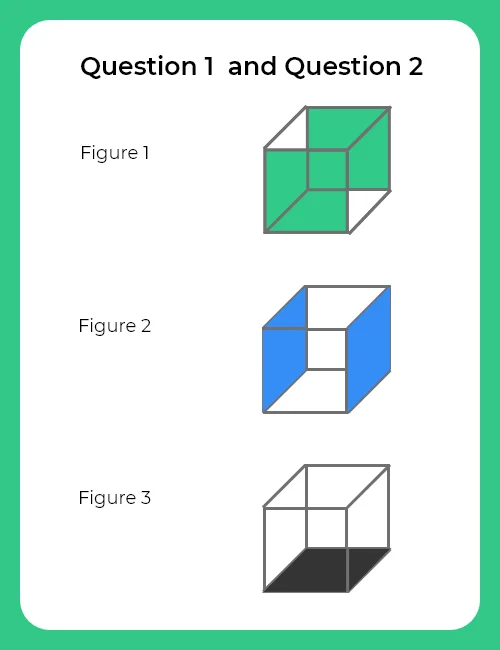# Formulas For Cube

## Formulas for Cube

In this Page Formulas for Cube is given. A cuboid having its length, breadth, height all to be equal in measurement is called as a cube.## Important Formulas for Cube

1. For a Cube of side n x n x n painted on all sides which is uniformly cut into smaller cubes of dimension 1 x 1 x 1,

• Number of cubes with 0 side painted = $(n-2) ^{3}$
• Number of cubes with 2 sides painted = $12(n-2)$
• Number of cubes with 1 sides painted = $6(n – 2) ^{2}$
• Number of cubes with 3 sidess painted = 8(always)

2. Rotation Formulas:

Single Rotations:

• Clockwise Rotation by 90 degrees (x-axis):
(x, y, z) -> (x, -z, y)
• Counterclockwise Rotation by 90 degrees
(x-axis):
(x, y, z) -> (x, z, -y)
• Clockwise Rotation by 90 degrees (y-axis):
(x, y, z) -> (z, y, -x)
• Counterclockwise Rotation by 90 degrees (y-axis):
(x, y, z) -> (-z, y, x)
• Clockwise Rotation by 90 degrees (z-axis):
(x, y, z) -> (-y, x, z)
• Counterclockwise Rotation by 90 degrees (z-axis):
(x, y, z) -> (y, -x, z)

Combined Rotations:

• Clockwise Rotation by 180 degrees (x-axis):
(x, y, z) -> (x, -y, -z)
• Clockwise Rotation by 180 degrees (y-axis):
(x, y, z) -> (-x, y, -z)
• Clockwise Rotation by 180 degrees (z-axis):
(x, y, z) -> (-x, -y, z)

3. Hidden Face Formula:
If you need to determine the face opposite a given face (when the cube is unfolded), you can use this formula:

Opposite Face of F = F ± 3

Where F is the face number (1 for front, 2 for right, 3 for back, etc.). The ± sign indicates that you can add or subtract 3 to get the opposite face.

4. Percentage Formula:
When calculating percentages related to cubes, use the formula:

Percentage =$\frac{Part}{Whole}\times100$

Where “Part” is the number of units in question (like the number of cells with a certain color or pattern), and “Whole” is the total number of units in the cube (total number of cells).

5. Counting Formula:
For counting specific attributes within a cube (like the number of cells with a particular color or pattern):

Count = Number of Units with Attribute

### Related Banners

Get PrepInsta Prime & get Access to all 200+ courses offered by PrepInsta in One Subscription

## Example for Cube Formulas

Question 1:
A cube having an edge of 12 cm each. It is painted green on two opposite faces, blue on one other pair of opposite faces, black on one more face and one face is left unpainted. Then it is cut into smaller cubes of 1 cm each.Find the total no. of smaller cubes
.

Solution:
Total number of Smaller cubes= $\frac{12\times 12\times 12}{1\times1\times 1}$ = 1728

Question 2.
The no. of smaller cubes which are having two-faces painted.

Solutions:
For 2 sides painted, we look for the edges.

A cube has 12 edges;
in which 8 edges each edge having 10 cubes will have 2 sides painted. (4 edges of an unpainted side won’t be included).
We’ll also include those 4 cubes (which we didn’t count while counting 3 colored sides, as they have 2 sides painted)
Cubes on 4 edges of the unpainted side of the cube will have 1 side painted (due to the unpainted side).

Therefore, total cubes with 2 sides painted= 8 x 10 + 4 = 84 cubes.## Get over 200+ course One Subscription

Courses like AI/ML, Cloud Computing, Ethical Hacking, C, C++, Java, Python, DSA (All Languages), Competitive Coding (All Languages), TCS, Infosys, Wipro, Amazon, DBMS, SQL and others